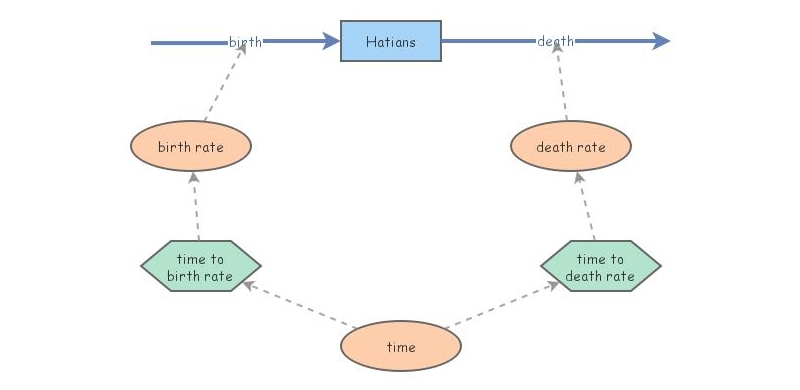Population of Haiti (Undeveloped) Over TimeHere we model the population of Haiti, a relativly underdeveloped country, given data between 1960 and 2013 from Worldbank.org. We used the crude birth rate and crude death rate for every 5 years since 1960 to 2005, and the rates every year from 2005 to 2013. To forecast, we used the slope of the net birth rate to calculate when the net birth rate would be zero, and used this year for our birth and death rates to are equal to zero. We assumed no net movement of people into or out of Haiti.